﻿ Driver Model Based on Mechanical Traveling Wave Principle

Driver Model Based on Mechanical Traveling Wave Principle

SONG Rui-Yin, LI Yue, ZHOU Ming-Long, LIN ZuanOPEN ACCESSPEER-REVIEWED

Driver Model Based on Mechanical Traveling Wave Principle

SONG Rui-Yin1,, LI Yue1, ZHOU Ming-Long1, LIN Zuan1

1Ningbo Institute of Technology, Zhejiang University, Ningbo, China

Abstract

A new type of mechanical driver based on traveling wave transmission principle was put forward. The initial structure of the reducer was designed and the theoretical models such as drive ratio, output torque and output power were built up. An initial reducer was manufactured and some performance parameters were tested.The experiment results illustrate that the new type of reducer proposed has a large drive ratio feature, and the testing results accord with the theoretical model.

At a glance: Figures

123
Prev Next

• Rui-Yin, SONG, et al. "Driver Model Based on Mechanical Traveling Wave Principle." American Journal of Mechanical Engineering 2.6 (2014): 159-163.
• Rui-Yin, S. , Yue, L. , Ming-Long, Z. , & Zuan, L. (2014). Driver Model Based on Mechanical Traveling Wave Principle. American Journal of Mechanical Engineering, 2(6), 159-163.
• Rui-Yin, SONG, LI Yue, ZHOU Ming-Long, and LIN Zuan. "Driver Model Based on Mechanical Traveling Wave Principle." American Journal of Mechanical Engineering 2, no. 6 (2014): 159-163.

 Import into BibTeX Import into EndNote Import into RefMan Import into RefWorks

1. Introduction

The drive principle of a traveling wave has been used in the field of ultrasonic wave motors, and it is evident that a traveling wave would be produced when a particle oscillates up and down about a fixed point. It is evident that a traveling wave would be produced when a particle oscillates up and down about a fixed point, and the drive principle of traveling wave has been used in the field of ultrasonic wave motor [1, 2, 3, 4]. Here, a new type of reducer based on the drive principle of a traveling wave has been put forward. The traveling wave occurs when a particle vibrates with certain frequency producing traveling waves, and the output waves transmit in a medium correspondingly. Compared with the gear reducer, this driver has some merits such as simple structure, large drive ratio and small dimensions, which can be used in some special fields such as large drive ratio mechanism and MEMS. In this paper, the theory of mechanical traveling wave driver has been in troduced, the structure of the driver was built and some mechanical drive ratio experiments were performed.

2. Driving Principle of Mechanical Traveling Wave

Figure 1 shows the theoretical model of mechanical traveling wave driver, which mainly includes the traveling wave producer, elastic layer and output layer. When the equally distributed rollers move, they tow and squeeze the elastic layer, and the particles of elastic layer move toward the other side to and fro, the particles then recover when the elastic layer is out of touch with the rollers. The periodic motion of stretch-press-stress makes the particles of elastic layer move in vertical vibration periodically. Combining the compound movements of the horizontal direction movements with the rollers and the vertical direction movements of particles, the mechanical traveling wave occured.

In the figure, the motion trail of the particle of the elastic layer is elliptical [4, 5, 6, 7, 17, 18]. When traveling wave moves toward the right, the movement direction of the particles is counter clock wise. When the particles arrive at the wave peak, the direction of the vibration velocity is toward the left. Under the condition of preload between elastic layer and output layer, the horizontal movement of the particles causes friction force on the output layer, driving the output layer movement towards the left and vice versa.

The shape of the motion trail for the elastic particles is an ellipse (shown in Figure 1). Point P0 is the initial position of the particle before bend deformation of the elastic layer and point P is another position of that point after bending deformation,is the bend angle between P and P0 and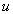is the vibration displacement along x direction. his the thickness of un-deformed elastic layer and a rectangular coordinate system can be built up along neutral surface of elastic layer. The equation of traveling wave for neutral surface is as follows [8, 9, 11, 12]: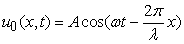(1)

where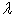is the wavelength of traveling wave for elastic layer

Then, the vibration w(x,t) displacement of particle from point P0 to P is as follows:(2)

Through a method of partial differential equations, the vibration velocity(vx) for particles of the elastic layer is as follows:(3)

A negative sign means that the motion direction of the particle is opposite to that of the motion direction for the traveling wave. If we do not consider the sliding effect between elastic layer and output layer, the output layer velocity(vF) equals the vibration velocity of the elastic particle at the bending wave crest location.(4)

Velocity curve of output layer is shown in Figure 2. The figure illustrates that only a half cycle of the velocity is useful for the movement of output layer. Without taking the influence of relative sliding into account, by calculating the dashed area of velocity curve shown in the figure. The mean velocity of output layer is given by: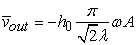(5)

3. Structure of Mechanical Traveling Wave Driver

Based on the theory of mechanical traveling wave drive, a mechanical traveling wave driver of a rotary type was designed. Figure 3 shows the structure of the driver, which includes such components as input shaft, output shaft and traveling wave producing roller etc. In the driver, a series of cylindrical balls are distributed equally along the input shaft. Mechanical traveling wave would be produced on the condition of cylindrical rollers rotating around input shaft, and the output shaft rotates correspondingly based on a traveling wave principle. The key part of the driver is the traveling wave producer, which includes an elastic layer and cylindrical rollers uniformly distributed along the input shaft (shown in Figure 3a). Traveling wave producer can be designed as of several shapes such as circular conical shape, torus shape and disk shape. Cross-section shape of traveling wave producer is shown in Figure 3 (b).

4. Modeling for Mechanical Traveling Wave Producer

4.1. Drive Ratio of Mechanical Traveling Wave Producer

Figure 4 shows the relationship between parameters for input shaft, traveling wave producer and output shaft. R1, R2, r, r1 and h represent distance between input shaft center and elastic layer center, input shaft radius, output shaft radius, curve radius between elastic layer and cylinder ball, radius of cylinder roller and the thickness of elastic layer respectively. Consider that the deformation of the elastic layer is small and that it can be ignored. Then the wave length of mechanical travelling wave driver is as follows:while T (wave period) is: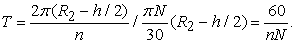We have:. Combined with Equation(5), the average velocity of output shaft is obtained as follows: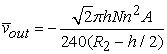(6)

Evidently, the biggest squeeze deformation of elasticlayer is the amplitude of the elastic layer, i.e. r1=A. The velocity of center part for elastic layer is obtained as:(7)

Setting total preload between input shaft and output shaft as F, the number of rollers is n, and the preload for single roller is: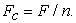The contact area between roller and the elastic layer is as follows: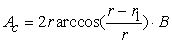(8)

where, r1(r1=A) is the deformation depth of the elastic layer, E is elastic modulus of the elastic layer and B is length of the cylindrical roller.

Consider that the value of the deformation depth of elastic layer(r1) is much smaller than that of the cylinder roller(r), the stress of the elastic layer is as follows: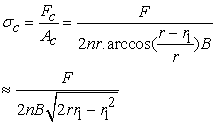(9)

In a traveling wave length period, the strain of elastic layer is given by [8, 9]:where, 2L is the contact length in a wavelength between input shaft and output shaft. Consider that the strain is produced in half of the wavelength period. The average value of the valid strain is as follows: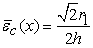(10)

Based on stress-strain relation formula: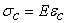, the relationship between squeeze deformation and preload is as follows:Because r1<<r, by simplifying the formula, the relationship between r1 and F (preload) is given by:(11)

Then, the total drive ratio is as follows: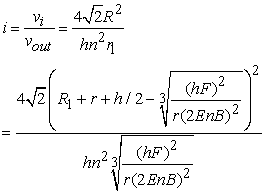(12)

It is obvious that the total drive ratio is influenced by such parameters as thickness of elastic layer, elastic layer materials, preload, radius of input (output) shaft, number of cylindrical rollers, radius of cylindrical rollers and amplitude etc.

4.2. Output Power for Mechanical Traveling Wave Producer

Friction layer between the elastic layer and output shaft is the key part to drive the output shaft. Considering the stiffness of elastic layer is larger than that of friction layer, it is supposed that friction layer hasdeformed while elastic layer has no deformation, and the elastic layer has the waveform shape. Considering theory of shearing deformation and Coulomb friction law, a contact model between the elastic layer and friction layer has been built, which is shown in Figure 5. In the figure, the interval [-xr,-xr1] is the viscous region, point (-xr1) is the demarcation point of viscous region to sliding friction region. Considering the influence of the viscous region, the effective area between the input layer and output layer decreases, and the output torque correspondingly decreases. Driving force from between input shaft and output shaft is given by [9, 10]: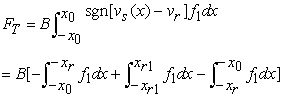(13)

where, B is the contact width of roller between input shaft and output shaft, f1 is the horizontal force between input shaft and output shaft, and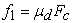,is the dynamic friction coefficient and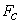is the preload.

It is difficult to accurately measure some parameters such asbecause the viscous region and sliding region are variable with materials and preload change. So, the equation (13) can be simplified as follows:(14)

where,is constant, which reflects the friction value. The average output torque of the producer is as follows: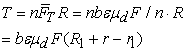(15)

Then, the output power is given by: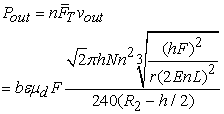(16)

5. Testing Device Experiment

A testing device with traveling wave principle was manufactured, which is shown in Figure 6. In the device, the kinematic chain consists of the electromotor, input shaft, mechanical traveling wave producer and output shaft. Rotational speed is measured by a speed sensor and displacement was measured by a linear scale sensor. Here, a straightedge was used as the output link instead of the output shaft. When the mechanical traveling wave was produced on the elastic layer, the straightedge would move toward a certain direction, and the displacement value was measured by a linear scale sensor. A feed screw nut device was used as the preload adjuster, and the preload can be adjusted by rotating the nut. The material used for the elastic layer is important, as it is a key part of the mechanical traveling wave producer. Here, PU (Polycarbamate) was selected as the initial material used for the elastic layer, and the thicknesses of the elastic layers was 3mm, 5mm, 7mm and 9mm, respectively.

According to Equation (12), we know that the total velocity ratio value is dependent on such parameters as thickness of elastic layer, material of elastic layer, amplitude of preload, radius of input and output shaft, number of rollers, radius of roller and amplitude etc. In the experiment, several parameters such as material of elastic layer, radius of input and output shaft and radius of roller were set as constants, while such parameters as thickness of elastic layer, number of rollers and preload were set as variables. In the testing device, the radius of the input shaft was 20mm and the angular velocity was 136r/min; the radius of the cylindrical roller was 1.8mm and the length of roller was 9mm, which were equally distributed along input shaft length, the diameter of the elastic layer is 48.16mm. Here, experiments on total drive ratio for the device by adjusting such parameters as thickness of elastic layer, number of cylinder rollers and preload were done.

5.1. Experiment through Adjusting the Thickness of the Elastic Layer

To test the influence of the thickness of the elastic layer vs. total drive ratio, several elastic layers with a thickness of 3mm, 5mm, 7mm and 9mm were studied. The preload between the elastic layer and the straightedge was changed by rotating the screw nut, and four displacement curves are shown in Figure 7. The velocity of the straightedge is almost constant, and the larger drive ratio is about 401.7. Secondly, the displacement value of the straightedge is from 189mm to 150mm. With the increase of thickness for the elastic layer, it was revealed that the thickness has an unobvious effect on the total drive ratio.

Figure 7. The displacement of output straightedge on condition of different elastic layer thicknesses (a-3mm, b-5mm, c-7mm, d-9mm)

In the experiment, the preload between elastic and straightedge was adjusted by rotating the nut of the screw rod, and the adjusted distance was 0.5mm. Here, two elastic layers with thicknesses of 3mm and 5mm were used in the experiment.

The curve of the drive ratio VS the deformation of the elastic layer is shown in Figure 8. we know that with the increase of preload (i.e. the increase of deformation for elastic layer) between the elastic layer and the straightedge, the total drive ratio decreased in a degree correspondingly, and the total drive ratio decreased with the thickness of elastic layer increase, which corresponds to the theoretical model of the total drive ratio.

5.3. Experiment through Adjusting Roller Number

The number of rollers is one of the key factors in a mechanical traveling wave producer. In the experiment, the numbers of rollers were set to 6,8,12 and 16, and the preload was constant. The curve of total drive ratio VS the rollers number is shown in Figure 9, which has exponential profile approximately. This illustrates that the total drive ratio decreases with the increase of roller numbers, which is consistent with the theoretical model.

6. Conclusions

We found that the total drive ratio model and the output power model of the traveling wave producer were built up, and the testing device was built correspondingly. Through a series of tests, some merits were drawn as follows: big drive ratio and simple structure is desirable.

Considering that the material of elastic layer and friction layer have an obvious influence on mechanical behavior of the device, a more accurate model needs to be established through the method of micro friction principle. Furthermore, it is indicated that the device has low efficiency, which needs to be improved by theory and structure methods.

References

  Diwakar, S. V.; Tiwari, Shaligram; Das, Sarit K.; Sundararajan, T. Stability and resonant wave interactions of confined two-layer Rayleigh-Benard systems [J].Journal of Fluid Mechanics. 2014, 754: 415-455.In article CrossRef  Ueha S. Tomikawa Y. Ultrasonic motors theory and applications[M]. Oxford science Publication, 1993.In article  P. LeMoal, P.Cudsin. Optimization of traveling wave ultrasonic motors using a three-dimension analysis of the contact mechanism at the stator rotor interface, Eur.J.Mech.A/solids, 1999, 18: 1061-1084.In article CrossRef  Yang, Lin; Zhao, Chunsheng. Flexible supporting and fixing method for hybrid ultrasonic motor using longitudinal and torsional vibration modes [J] JOURNAL OF VIBROENGINEERING. 2014.16(9): 2854-2861.In article  Glenn, T.S., Ghandi, K.; Atalla, M.J.; Hagood, N.W. Mixed-domain Traveling-wave Motor Model with Lossy (complex) Material Properties[C]: Proceedings of SPIE - The International Society for Optical Engineering, 2001, 4326: 525-537.In article  Hirata, Hiroshi; Ueha, Sadayuki. Design of a Traveling Wave Type Ultrasonic Motor[C]: IEEE Transactions on Ultrasonics, Ferroelectrics, and Frequency Control, 1995:v 42(2): 225-231.In article CrossRef  Hagood N W, Mcfarl and A J. Modeling of a piezoelectric rotary ultrasonic motor[J]. IEEE LJFFC. 1995. 42(2): 210-224.In article  Takashi Maneo, Takayuki Tsukimoto, Akira Miyake. Finite element analysis of the motor/stator contact in a ring-type ultrasonic motor[J]. IEEE Trans on UFFC, 1992, 39(6):668-674.In article CrossRef PubMed  Wang Ping; ones, L. S.; YangQun; Gurupackiam, S. Cell transmission model based traffic signal timing in oversaturated conditions[J]. Journal of Central South University. 2013, 20(4)1129-1136.In article CrossRef  Shen, Shengnan; Lee, Heow P.; Lim, Siak P. Three-dimensional finite element analysis of interfacial delamination in traveling wave ultrasonic motors[J]. 2014,23(7): 964-978.In article  Li Huafeng, Zhao ChunshengGuChenglin.Contact Model of Traveling Wave-type Ultrasonic Motor[J]. J. HuazhongUniv of Sci. & Tech. ( Nature Science Edition). 2004. 32(10)57-59.In article  Kurosawa, Minoru Kuribayashi.Ultrasonic linear motor using traveling surface acoustic wave[C]:2009 IEEE International Ultrasonics Symposium and Short Courses: 1096-1105.In article  Zou, G. B, Gao, H. L. Extra high speed hybrid protection scheme for high voltage transmission line[J]. International Journal of Electrical Power & Energy Systems. 2014. 63(5)83-90.In article CrossRef  Wang, Shiyu; Xiu, Jie; Cao, Shuqian. Analytical Treatment With Rigid-Elastic Vibration of Permanent Magnet Motors With Expanding Application to Cyclically Symmetric Power-Transmission Systems[J]. Journal of Vibration and Acoustics-transactions of the ASME. 2014. 136(2).In article CrossRef  Alijani, Farbod; Amabili, Marco. Nonlinear vibrations and multiple resonances of fluid filled arbitrary laminated circular cylindrical shells [J].Composite Structures. 2014. 108(2)951-962.In article CrossRef  Kim, Heuicheol; Cho, Mi-Gyung; Park, Jaehong; Bai, Cheolho; Shim, Jaesool. Prediction of gas pulsation of an industrial compressor[J].Journal of Central South University. 2013, 20(10) 2735-2740.In article CrossRef  Wang, Tianshi; Lancee, Charles; Beurskens, Robert. Development of a high-speed synchronous micro motor and its application in intravascular imaging [J]. SENSORS AND ACTUATORS A-PHYSICAL.2014, 208(10)60-68.In article  Monti, Chiara; Vetrella, Ugo Besi; Mugnaini, Giampiero. Test of piezo-ceramic motor technology in ITER relevant high magnetic fields[J]. FUSION ENGINEERING AND DESIGN. 2014, 89(10) 2304-2308.In article CrossRef